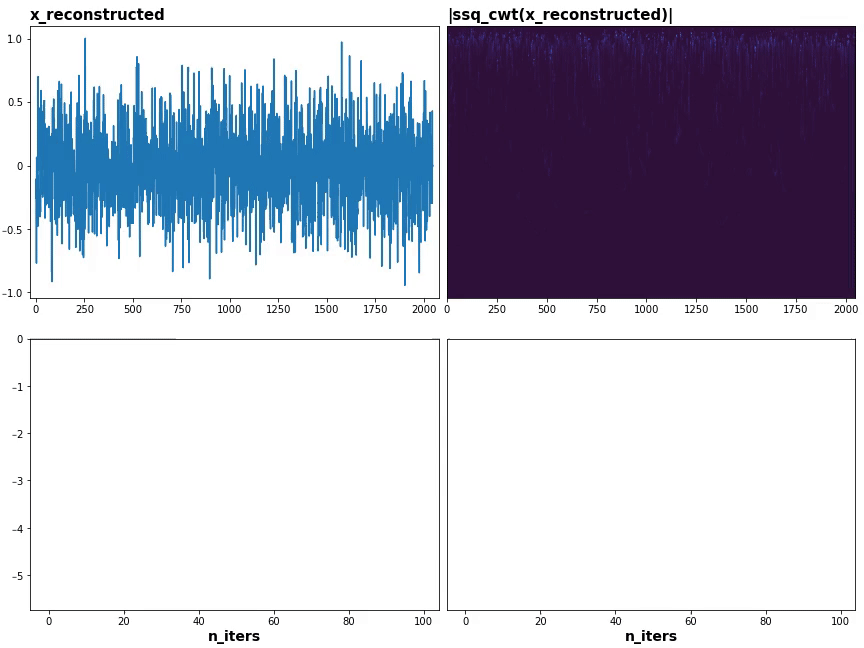# Inverting a scalogram

A scalogram is said to discard phase information with modulus; is it still possible to recover the signal to some extent?

Algorithms like Griffin-Lim can invert within an error, but what's the theoretical bound (ideal case) on accuracy? Are there better approaches?

Algorithms aside, a scalogram is proven to be strongly invertible - perfectly for recovering instantaneous frequency and amplitude; see "Invertibility". Besides Griffin-Lim and alike, since CWT is fully differentiable, we can use gradient-based reconstruction - and it should outperform handcrafted algorithms with proper tuning.

Hard part's ensuring every involved operation is differentiable; this is automated with PyTorch and TensorFlow, as long as using their ops. The train loop is trivial:

1. Compute $$S(x)$$
2. Initialize $$x_\text{rec}$$ randomly
3. Compute $$S(x_\text{rec})$$
4. Compute loss, e.g. MSE: $$\sum|S(x_\text{rec}) - S(x)|^2$$
6. Update $$x_\text{rec}$$ with gradients

PyTorch example with Kymatio:

import torch, kymatio

sc = kymatio.Scattering1D(shape=2048, J=6, Q=8, frontend='torch')
x = torch.cos(40*torch.linspace(0, 1, 2048))
Sx = sc(x)

xrec = torch.randn(len(x))
optimizer = torch.optim.SGD([xrec], lr=500, momentum=.9)
loss_fn = torch.nn.MSELoss()

for i in range(100):
Sxrec = sc(xrec)
loss = loss_fn(Sxrec, Sx)
loss.backward()
optimizer.step()


• Learning rate decay
• Swapping L2 to L1 past certain loss, to emphasize small deviations
• Coefficient renormalization (overall Gaussianization and as described in VI. B)

### Visualizing

Plot the recovered signal and its scalogram at each gradient iteration; since synchrosqueezed is more informative if we know the ridges, I'll use it instead:A close approximation is attained in about 20 iterations.

Code available at Github.